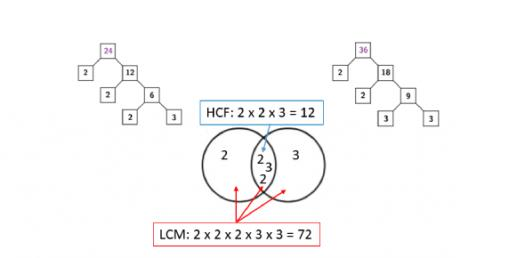# Shortcut Of LCM And HCF Quiz

10 Questions | Total Attempts: 448SettingsLCM and HCF are concepts useful in mathematics as well as in determining the time and distance, pipes and cisterns and so on. There are many shortcuts and tricks in analyzing the concepts problems which requires some principles and methods for easy calculations. Co-prime numbers, Prime numbers and terms like multiples, factors and so on are required for easy use of the shortcuts.

• 1.
What is the least common multiple of 3 and 4?
• A.

24

• B.

12

• C.

48

• D.

36

• 2.
What is the integral values of number that can provide the number?
• A.

Prime numbers

• B.

LCM

• C.

HCF

• D.

Prime Factors

• 3.
Which of these always divides the LCM of a number?
• A.

10

• B.

Prime Factors

• C.

HCF

• D.

LCF

• 4.
Which of these is the multiple of 3 and 5?
• A.

15

• B.

10

• C.

12

• D.

14

• 5.
What is the other name for HCF?
• A.

LCF

• B.

GCF

• C.

HCM

• D.

PCM

• 6.
What is the name given to number having many factors?
• A.

Even number

• B.

Odd number

• C.

Composite number

• D.

Prime number

• 7.
What is the product of common prime factors in respect to highest indices?
• A.

1

• B.

GCF

• C.

HCF

• D.

LCM

• 8.
What is the common multiple of 2,3,6 and 9?
• A.

9

• B.

16

• C.

18

• D.

20

• 9.
Which of these is not a prime number?
• A.

3

• B.

5

• C.

17

• D.

4

• 10.
Which of these numbers are said to be co-primes?
• A.

1 and 2

• B.

1 and 4

• C.

2 and 3

• D.

6 and 7

Related TopicsBack to top